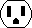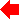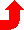Chapter 9: Tools9-5: Simulation (built-in) 9-5-6: ALS ModelsAs previous examples have shown, the model entity provides connectivity between other entities, including other model entities. The model may be used in conjunction with gate and function entities to describe the behavior of any circuit.

The model entity is headed by a model declaration statement and followed by a body which references instances of other entities, lower in the hierarchy. The model name and a list of exports (which are referenced in a higher level model description) are included in this statement. The format of the model declaration statement is:

 Format: model name(signal1, signal2, signal3, ... signalN) Example: model dff(d, ck, set, reset, q, q_bar)

References to instances of primitive objects (gates and functions) and lower level models are used to describe the topology of the model to the simulator. The format of an instance reference statement is:

 Format: instance : model ( signal1, signal2, signal3, ... signalN ) Example: gate1: subgate(input, en, mix)

It should be noted each instance reference in a model entity must have a unique instance name. The following is an example of the use of a model entity:

 ``` model latch(input, en, en_bar, out)    gate1: xgate(input, en, mix) gate2: xgate(out, en_bar, mix) gate3: inverter(mix, out_bar) gate4: inverter(out_bar, out) ``` ``` gate xgate(in, ctl, out) t: delta=8.0e-9 t: delta=8.0e-9 i: ctl=L o: out=X@0 i: ctl=H in=L o: out=L i: ctl=H in=H o: out=H i: o: out=X@2 gate inverter(in, out) t: delta=5.0e-9 i: in=L o: out=H i: in=H o: out=L i: o: out=X@2 ```

This example contains the description of a simple latch. When the enable signal is asserted high (en=H, en_bar=L) the input data passes through the transmission gate (gate1) and then through two inverters where it eventually reaches the output. When enable is asserted low (en=L, en_bar=H) the input connection is broken and the feedback transmission gate (gate2) is turned on.

#### The Set Statement

The set statement is used to initialize signals in the model description to specific logic states before the simulation starts. This feature is useful for tying unused inputs to power(H) or ground(L).PreviousTable of Contents Next